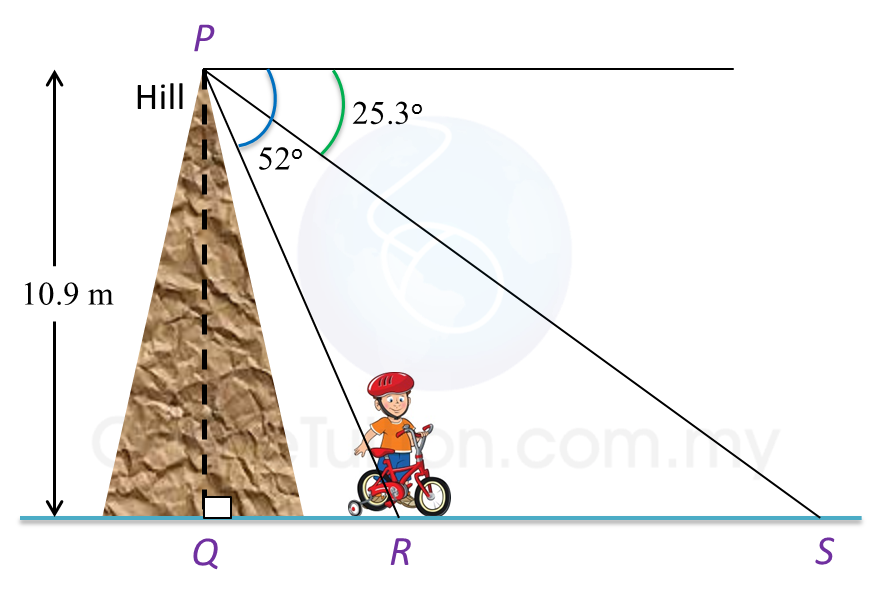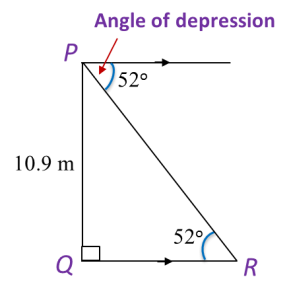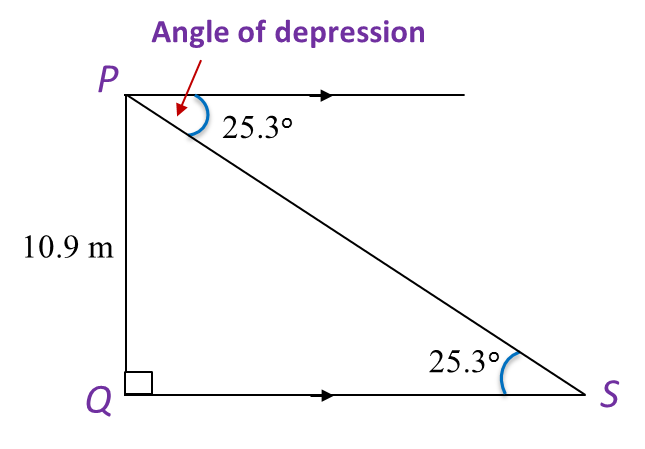# 10.2 Solving Problems Involving Angles of Elevation and Depression

Example:
The angle of depression of a cycling kid measured from a hill with 10.9 m high is 52o. When the kid cycles along the hillside and stops, the angle of depression becomes 25.3o. What is the distance cycled by the kid along the hillside?

Solution:Step 1: Draw a diagram to represent the situation.
Step 2: Devise a plan.
Find the lengths of QS and QR. Then, QS – QR = distance cycled by the kid.$\begin{array}{l}\mathrm{tan}{52}^{o}=\frac{10.9}{QR}\\ QR=\frac{10.9}{\mathrm{tan}{52}^{o}}\\ QR=8.5m\end{array}$$\begin{array}{l}\mathrm{tan}{25.3}^{o}=\frac{10.9}{QS}\\ QS=\frac{10.9}{\mathrm{tan}{25.3}^{o}}\\ QS=23.1m\end{array}$

QS – QR = (23.1 – 8.5) m = 14.6 m

Therefore the kid has cycled 14.6 metres.